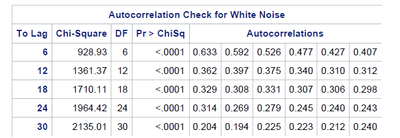## ACF using Proc ARIMA

Hi,

I want to find the autocorrelation of a time series variable for different orders (e.g., AC(1), AC(5), AC(20)), so I use the following code:

proc arima data=mydata plot(only)=(series(corr)) ;
identify var=tsvar nlag=20;
run;

I have two questions regarding the following table in the output:1. How could I determine the order of autocorrelation, so instead of 6,12, etc, it'll give me the result for 1,5, etc.

2. Under Autocorrelations, there are six columns. SAS manual says "The autocorrelations are checked in groups of six". what does it mean in groups of six?

Thanks,

1 ACCEPTED SOLUTION

Accepted Solutions

## Re: ACF using Proc ARIMA

Hi @DavyJones ,

@Ksharp 's explanation and your understanding are correct.  Based on the table you provided, the values in the "Autocorrelations" portion of the "Autocorrelation Check for White Noise" table contain the autocorrelation coefficients at the individual lags.  For example, the first row in the table contains the autocorrelation coefficients at lag1, lag2, ...lag6.  In other words, the fifth value in that row, 0.427, is the autocorrelation coefficient at lag 5.  The second row contains the autocorrelation coefficients for lags 7, 8, 9,...12.  The third value in the second row, 0.375, is the autocorrelation coefficient at lag 9.  If you add the OUTCOV= option to the IDENTIFY statement, you can create a data set with the autocorrelations in a format that you might prefer.  For example:

``````proc arima data=mydata plot(only)=(series(corr)) ;
identify var=tsvar nlag=20 outcov=mycorr;
run;

proc print data=mycorr;
run;``````

Regarding your second question, the Chi-Square statistic that is printed on each row is the Ljung-Box statistic.  It is used to test the null hypothesis that the series is white noise (ie. no autocorrelation).  These test statistics are computed by using sets of autocorrelation coefficients, therefore, the first Chi-Square statistic and associated DF and Pr>ChiSq values are based on the set of the first 6 autocorrelations.  The Chi-Square statistic and its associated DF and p-value in the second row are based on the set of the first 12 autocorrelations, etc.

If you want to either obtain a test of significance on each individual autocorrelation coefficient or compute the Ljung-Box test statistic using autocorrelations up through lag "p", then you can use the TIMESERIES procedure.  Please see the following code for an example:

``````proc timeseries data=mydata outcorr=corr plots=corr;
var tsvar;
corr lag n acf acfprob wn wnprob /nlag=30;
run;

proc print data=corr;
run;``````

For additional details on the calculations performed by the CORR statement, please see the following documentation link:

I hope this helps!

DW

4 REPLIES 4Ksharp
Super User

## Re: ACF using Proc ARIMA

If I was right . from left to right should be

ToLage Autocorrlation
6 Lag1 Lag2 .......Lag6

## Re: ACF using Proc ARIMA

Thanks! Does it mean that when To Lag=12, those six columns are going to be lag7, lag8, ..., lag12?Ksharp
Super User

## Re: ACF using Proc ARIMA

Yes . I think so.
Hope some experts to confirm it.
Or check SAS Documentation of proc arima .

## Re: ACF using Proc ARIMA

Hi @DavyJones ,

@Ksharp 's explanation and your understanding are correct.  Based on the table you provided, the values in the "Autocorrelations" portion of the "Autocorrelation Check for White Noise" table contain the autocorrelation coefficients at the individual lags.  For example, the first row in the table contains the autocorrelation coefficients at lag1, lag2, ...lag6.  In other words, the fifth value in that row, 0.427, is the autocorrelation coefficient at lag 5.  The second row contains the autocorrelation coefficients for lags 7, 8, 9,...12.  The third value in the second row, 0.375, is the autocorrelation coefficient at lag 9.  If you add the OUTCOV= option to the IDENTIFY statement, you can create a data set with the autocorrelations in a format that you might prefer.  For example:

``````proc arima data=mydata plot(only)=(series(corr)) ;
identify var=tsvar nlag=20 outcov=mycorr;
run;

proc print data=mycorr;
run;``````

Regarding your second question, the Chi-Square statistic that is printed on each row is the Ljung-Box statistic.  It is used to test the null hypothesis that the series is white noise (ie. no autocorrelation).  These test statistics are computed by using sets of autocorrelation coefficients, therefore, the first Chi-Square statistic and associated DF and Pr>ChiSq values are based on the set of the first 6 autocorrelations.  The Chi-Square statistic and its associated DF and p-value in the second row are based on the set of the first 12 autocorrelations, etc.

If you want to either obtain a test of significance on each individual autocorrelation coefficient or compute the Ljung-Box test statistic using autocorrelations up through lag "p", then you can use the TIMESERIES procedure.  Please see the following code for an example:

``````proc timeseries data=mydata outcorr=corr plots=corr;
var tsvar;
corr lag n acf acfprob wn wnprob /nlag=30;
run;

proc print data=corr;
run;``````

For additional details on the calculations performed by the CORR statement, please see the following documentation link:

I hope this helps!

DW

Discussion stats
• 4 replies
• 570 views
• 2 likes
• 3 in conversation